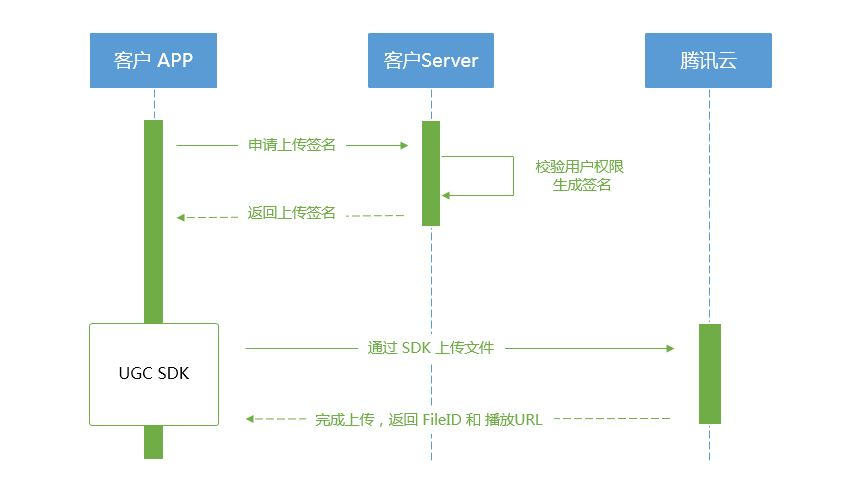## 总体介绍• 客户端首先向签名派发服务请求上传签名。
• 签名派发服务校验该用户是否有上传权限，若校验通过，则生成签名并下发；否则返回错误码，上传流程结束。
• 客户端拿到签名后使用短视频 SDK 中集成的上传功能来上传视频。
• 上传完成后，点播后台会发送 上传完成事件通知 给开发者的事件通知接收服务。
• 如果签名派发服务在签名中指定了视频处理 任务流，点播服务会在视频上传完成后根据指定流程自动进行视频处理。短视频场景下的视频处理一般为 AI 鉴黄
• 视频处理完成之后，点播后台会发送 任务流状态变更事件通知 给开发者的事件通知接收服务。

## 签名派发服务实现示例

``````/**
* 计算签名
*/
function createFileUploadSignature({ timeStamp = 86400, procedure = '', classId = 0, oneTimeValid = 0, sourceContext = '' }) {
// 确定签名的当前时间和失效时间
let current = parseInt((new Date()).getTime() / 1000)
let expired = current + timeStamp;  // 签名有效期：1天
// 向参数列表填入参数
let arg_list = {
//required
secretId: this.conf.SecretId,
currentTimeStamp: current,
expireTime: expired,
random: Math.round(Math.random() * Math.pow(2, 32)),
//opts
procedure,
classId,
oneTimeValid,
sourceContext
}
// 计算签名
let orignal = querystring.stringify(arg_list);
let orignal_buffer = new Buffer(orignal, "utf8");
let hmac = crypto.createHmac("sha1", this.conf.SecretKey);
let hmac_buffer = hmac.update(orignal_buffer).digest();
let signature = Buffer.concat([hmac_buffer, orignal_buffer]).toString("base64");
return signature;
}
/**
* 响应签名请求
*/